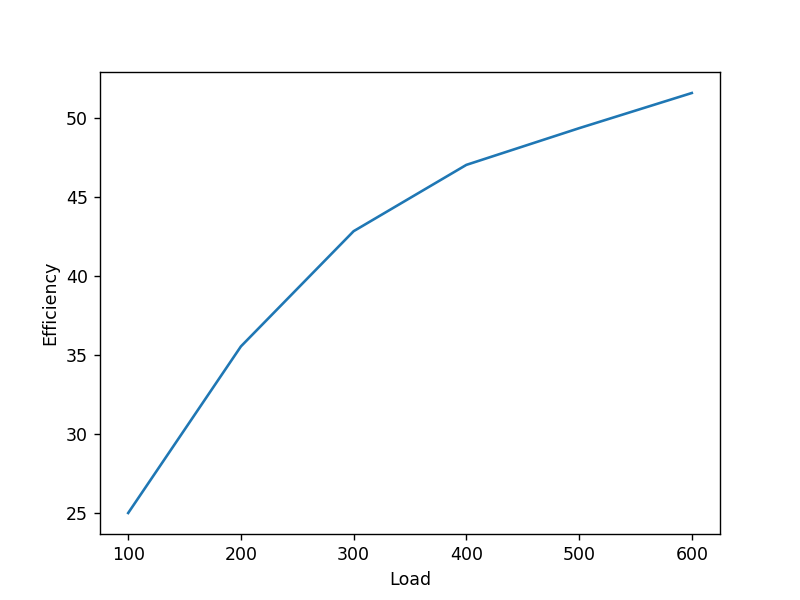Example 6.1¶

In :
import math
P = 500.0    #Effort
D = 20.0   #Distance moved by the effort
d = 0.8     #Distance moved by the load
VR=D/d      #Velocity Ratio
Efficiency=MA/VR
Pi =W/VR        #Ideal effort
efl=P-Pi            #Effort lost in friction
Fr=Wi-W            #frictional resistance
print "Velocity Ratio",VR
print "Efficiency",Efficiency
print "Ideal Effort",Pi
print "Effort lost in friction",efl
print "frictional resistance",Fr
Velocity Ratio 25.0
Efficiency 0.8
Ideal Effort 400.0
Effort lost in friction 100.0
frictional resistance 2500.0

Example 6.2¶

In :
import math
P1= 150.0    #Effort1

P2= 180.0    #Effort2
P3= 200.0    #Effort3
#law of machine is given by P=mW+C
m=(P2-P1)/(W2-W1)
C=P2-m*W2
print "Law of machine is P=",m,"W","+",C
VR=30.0      #Velocity Ratio
Efficiency=MA/VR*100
Pi =W3/VR        #Ideal effort
print "Ideal effort is",Pi,"N"

efl=P3-Pi            #Effort lost in friction

print "Effort lost in friction",efl
print "Efficiency",Efficiency
Law of machine is P= 0.05 W + 30.0
Ideal effort is 113.333333333 N
Effort lost in friction 86.6666666667
Efficiency 56.6666666667

Example 6.3¶

In :
import math
P1= 150.0    #Effort1
Efficiency=0.6
VR=MA/Efficiency        #Velocity Ratio
print "Velocity Ratio",VR
P2= 250.0    #Effort2
MA=W2/P2
Efficiency=MA/VR*100
print "Efficiency",Efficiency
#law of machine is given by P=mW+C
m=(P2-P1)/(W2-W1)

MaxEfficiency=MMA/VR*100

print "Maximum Efficiency",MaxEfficiency
Velocity Ratio 85.5555555556
Efficiency 61.7142857143
Maximum Efficiency 64.2857142857

Example 6.4¶

In :
%matplotlib notebook
import matplotlib
import numpy as np
import matplotlib.pyplot as plt2
P=[16.0,22.5,28.0,34.0,40.5,46.5]    #Efforts
VR=25.0             #velocity ratio
E=[0,0,0,0,0,0]  #Efficiency
#calculating average slope
m=(P-P)/(W-W)
C=P-m*W
print "Law of machine is P=",m,"W","+",C
for i in range(0,6):

E[i]=W[i]/(25*P[i])*100                     #E=W/(P*VR)

plt2.plot(W,E)
plt2.ylabel("Efficiency")
plt2.show()

MaxEfficiency=1/VR*100*1/m

print "Maximum Efficiency",MaxEfficiency

Law of machine is P= 0.06 W + 10.5Maximum Efficiency 66.6666666667

Example 6.5¶

In :
P = 360.0    #Effort

VR=30.0      #Velocity Ratio
Efficiency=MA/VR*100.0
var="reversible machine"
if Efficiency < 50.0:
var="self-locking machine"

Fr=Wi-W            #frictional resistance
print "Velocity Ratio",VR
print "Efficiency",Efficiency
print var

print "frictional resistance",Fr
Velocity Ratio 30.0
Efficiency 46.2962962963
self-locking machine
frictional resistance 5800.0

Example 6.6¶

In :
import math
N=3.0              #number of pulleys
VR=2**N              #Velocity Ratio
L=0.05                     #Efficiency loss  in each pulley
Efficiency=0.8
P = W/MA    #Effort
print "Effort is",P,"N"
#second case
P=520.0
n=0,
for i in range(3,20):
if((P*(0.8-(i-3)*0.05)*(2**i)))>6000:
n=i
break

print "number of pulley is ",n
Effort is 937.5 N
number of pulley is  4

Exmple 6.7¶

In :
import math
N=3.0              #number of movable pulleys
VR=2*N              #Velocity Ratio
L=0.05                     #Efficiency loss  in each pulley
Efficiency=0.85
P = W/MA    #Effort
print "Effort is",P,"N"
Effort is 2352.94117647 N

Example 6.8¶

In :
import math
N1=2.0              #number of movable pulleys in  system 1
N2=2.0              #number of movable  puleys in system 2
VR=2*N1+2*N2              #Velocity Ratio
L=0.05                     #Efficiency loss  in each pulley
Efficiency=0.78
P = W/MA    #Effort
print "Effort is",P,"N"
Effort is 1923.07692308 N

Example 6.9¶

In :
import math
N=3.0              #number of pulleys
VR=2**N-1              #Velocity Ratio
P = 180.0    #Effort
Efficiency=MA/VR*100
print "Efficiency",Efficiency
Pi =W/VR        #Ideal effort

efl=P-Pi            #Effort lost in friction
print "Effort lost in friction",efl
Efficiency 79.3650793651
Effort lost in friction 37.1428571429

Example 6.10¶

In :
import math
N1=2.0              #number of movable pulleys in  system 1 in figure B
N2=2.0              #number of movable  puleys in system 2 in figure C
VR=2**N1-1+2**N2-1              #Velocity Ratio
Efficiency=0.70
P = W/MA    #Effort
print "Effort is",P,"N"
Effort is 595.238095238 N

Example 6.11¶

In :
D=500.0            #diameter of the wheel
d=200.0            #diameter of axle
tcw=6.0             #thickness of the cord on the wheel
tca=20.0           #thickness of the cord on the axle
W=1200              #effort
ED=D+tcw            #Effective diameter of the wheel
Ed=d+tca            #Effectivediameter of axle
VR=ED/Ed           #Velocity Ratio
print "Velocity ratio is ",VR
Efficiency=0.7
P = W/MA    #Effort
print "Effort is",P,"N"
Velocity ratio is  2.3
Effort is 745.341614907 N

Example 6.12¶

In :
D=800.0            #diameter of the wheel
d1=250.0            #diameter of axle 1
d2=300.0            #diameter of axle 2

W=20000.0              #effort

VR=(2*D)/(d2-d1)           #Velocity Ratio
print "Velocity ratio is ",VR
Efficiency=0.55
P = W/MA    #Effort
print "Effort is",P,"N"
Velocity ratio is  32.0
Effort is 1136.36363636 N

Example 6.13¶

In :
D=500.0            #diameter of the wheel
d=200.0            #diameter of axle

W=5000.0              #effort

VR=(2*D)/(D-d)           #Velocity Ratio
print "Velocity ratio is ",VR
Efficiency=0.6
P = W/MA    #Effort
print "Effort is",P,"N"
Velocity ratio is  3.33333333333
Effort is 2500.0 N

Example 6.14¶

In :
D=40.0  #Screw diameter
l=20.0   #Screw lwngth
W=40000.0              #effort
R = 400  #Lever length
u = 0.12    #coefficient of friction between screw and nut
P = (D/(2*R))*W*((u+(p/(3.14*D)))/(1-u*(p/(3.14*D))))   #Effort
print "Effort is",P,"N"
Effort is 348.376068376 N

Example 6.15¶

In :
import math
d=50.0  #mean diameter of screw
p=10.0  #pitch of screw
u=0.05 #coefficient of friction at the screw thread
R=300.0 ##Lever length
o1=math.atan(p/(3.14*d))
o2=math.atan(0.05)
P=d/(2*R)*(W*math.tan(o1+o2))  #effort
print "Effort is",P,"N"
VR=2*3.14*R/p       #Velocity Ratio
Efficiency=MA/VR*100.0
print "Efficiency",Efficiency,"%"
var="reversible machine"
if Efficiency < 50.0:
var="self-locking machine"
print var
T =d/2.0*W*math.tan(o1-o2) #The torque required to keep the load from descending
print "The torque required to keep the load from descending",T,"Nm"
Effort is 57.0287539936 N
Efficiency 55.8439936484 %
reversible machine
The torque required to keep the load from descending 2047.61904762 Nm

Example 6.16¶

In :
import math
p1=5.0   #Pitch of smaller screw
p2=10.0    #Pitch of larger screw
R=500.0     #Lever arm length from centre of screw
P=185.0    #Effort
VR=2*3.14*R/(p2-p1)       #Velocity Ratio
Efficiency=MA/VR*100.0

print "Efficiency",Efficiency,"%"
Efficiency 12.9110001721 %

Example 6.17¶

In :
d=200.0  #Diameter of the load drum
R = 1200.0   # Length of lever arm
T1 = 10.0  #Number of teeth on pinion,
T2 = 100.0   #Number of teeth on spur wheel
VR=R*T2/(d*T1)*2.0       #Velocity Ratio
print "Velocity Ratio is ",VR
P1= 100.0    #Effort1

P2= 160.0    #Effort2

#law of machine is given by P=mW+C
m=(P2-P1)/(W2-W1)
C=P2-m*W2
print "Law of machine is P=",m,"W","+",C
Efficiency=MA/VR*100.0

print "Efficiency for first case",Efficiency,"%"
Efficiency=MA/VR*100.0

print "Efficiency for second case",Efficiency,"%"
Velocity Ratio is  120.0
Law of machine is P= 0.01 W + 70.0
Efficiency for first case 25.0 %
Efficiency for second case 46.875 %

Example 6.18¶

In :
d=150.0  #Diameter of the load drum
R = 400.0   # Length of lever arm
T1 = 15.0  #Number of teeth on pinion,
T3 = 20.0  #Number of teeth on pinion,
T2 = 45.0   #Number of teeth on spur wheel
T4 = 40.0   #Number of teeth on spur wheel
P= 250.0    #Effort
Efficiency=0.4
VR=R*T2/(d*T1)*2.0*T4/T3       #Velocity Ratio
print "Velocity Ratio is ",VR